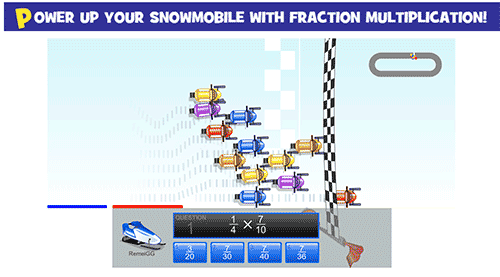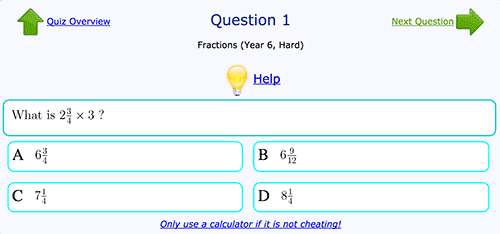## Friday, 13 January 2023

### Unit 4 - Multiplying fractions

Hello, hello!
We're finishing the unit on fractions so here you have the reminder on how to multiply fractions. It's SO easy!Here you have an explanation:How to multiply fractions in Mathsisfun
Now, I'll share some online exercises and games so you can practise further:Multiplying fractions gameSnowmobile game with fraction multiplicationMultiplying mixed fractions gameMultiplying fractions exercise - Easy levelMultiplying fractions exercise - Hard levelWho wants to be a hundredaire? game with multiplication of fractions
I hope you find this useful!
Bye, bye, butterflies! 🦋